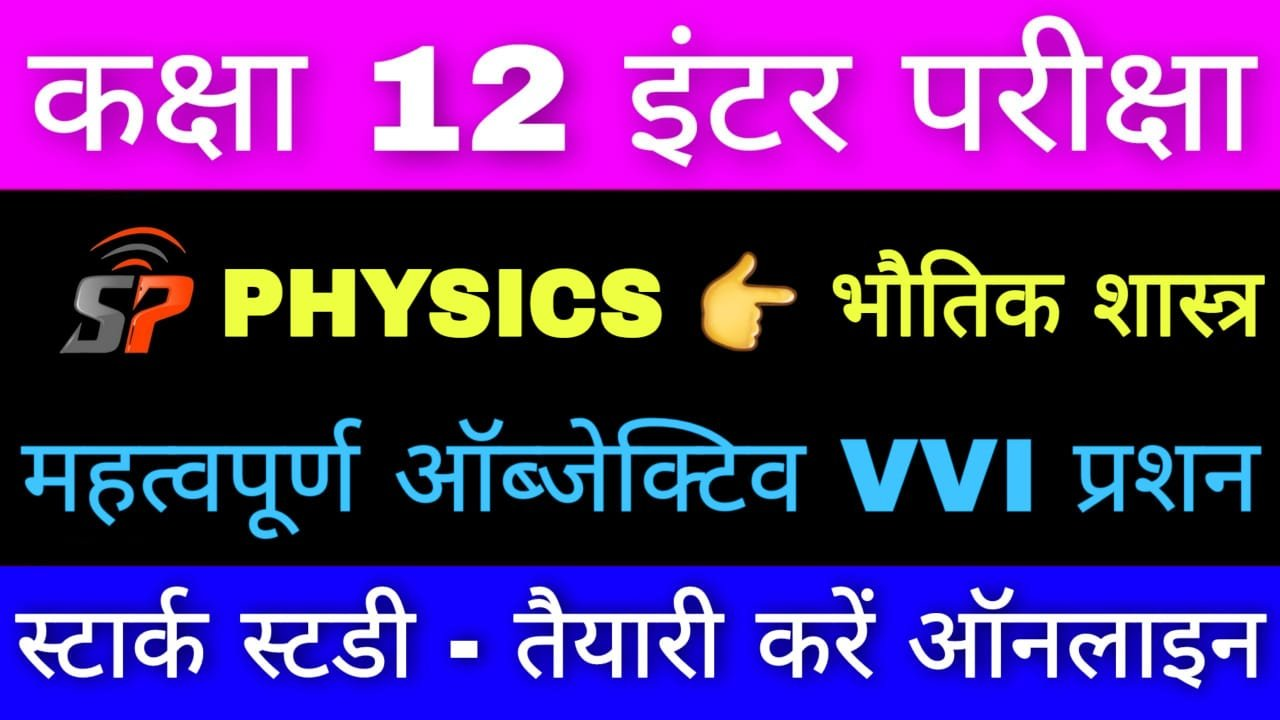Class 12th Physics Most VVI Objective Alternating Current Chapter

Alternating Current

1. In an LCR series ac circuit, the voltage across each of the components L, C and R is 50 Volt. The voltage across LC combination is :

(A) 50 Volt

(B) 25 Volt

(C) 100 Volt

(D) 0 Volt

2. The phase difference between current and voltage in an AC circuit is θ. Then power factor will be:

(A) cosθ

(B) sinθ

(C) tanθ

(D) 1 θ

3. The working principle of choke coil is based on

(A) Conservation of angular momentum

(B) Self induction

(C) Mutual induction

(D) Conservation of momentum

4. In a step up transformer the number of coils in primary coils is N1 and N2 in secondary. Then :-

(A) NI = N2

(B) NI < N2

(C) NI > N2

(D) None of these

5. The device that increases AC voltage is called –

(A) resistor

(B) step-down transformer

(C) step-up transformer

(D) transformer

6. The oscillation frequency of an LC circuit is f. If both the capacitance and inductance be doubled, the frequency will become –

(A) f / 4

(B) 2f

(C) 4f

(D) f / 2

7. In LCR-circuit, L = 8.0 H, C = 0.5 μF, and R = 100 Ω are connected in series. The resonant frequency will be

(C) 600 hertz

(D) 500 hertz

8. A choke coil is used to control current in a circuit of

(A) ac only

(B) dc only

(C) both ac and dc

(D) None of these

9. Step-down transformer increases

(A) current

(B) voltage

(C) wattage

(D) None of these

10. The thermal effect of alternating current is practically

(A) Joule heating

(B) Peltier heating

(C) Thomson effect

(D) None of these

11. The power factor of a choke coil is about

(A) 90°

(B) 0

(C) 1

(D) 180°

12. The power factor of a condenser is

(A) 90°

(B) 1

(C) 180°

(D) 0

13. For which of the following, capacitor works as infinite resistance ?

(A) DC

(B) AC

(C) both DC and AC

(D) None of these

14. L-C circuit without AC-source is called

(A) oscillatory circuit

(B) drift circuit

(C) hysteresis circuit

(D) None of these

15. S.I. unit of Impedance is

(A) henry

(B) ohm

(C) tesla

(D) None of these

16. The equation of an AC is I = 60sin100πt. The root mean square value will be

(A) 60√2

(B) 60 / √2

(C) 100

(D) zero

17. The value of phase difference between current and emf in an AC circuit with resistance only, is

(A) zero

(B) π / 2

(C) π

(D) 2π

18. The unit of reactance is

(A) ohm

(C) ampere

(D) mho

19. The unit of capacitive reactance is

(B) ohm

(C) maxwell

(D) ampere

20. The impedance of L-R series circuit is

(A) R = ωL

(B) R2 + ω2L2

(C) √R22L2

(D) R

21. In LCR circuit energy loss in circuit is

(A) in inductance

(B) in resistance

(C) in condenser

(D) All of above

22. The frequency of domestic electricity supply is 50 Hz. The frequency at which current becomes zero is

(A) 25

(B) 50

(C) 100

(D) 200

23. The frequency of alternating current supply in India is

(A) 50 hertz

(B) 60 hertz

(C) 100 hertz

(D) 220 hertz

24. The conversion of electrical energy into thermal energy in a transformer is called

(A) copper loss

(B) iron loss

(C) hysterisis loss

(D) None of these

25. In A.C. circuit, the current and voltage are given by i = 5 cos wt and V = 200sin wt respectively. Power loss in the circuit

(A) 20W

(B) 40W

(C) 1000W

(D) zero